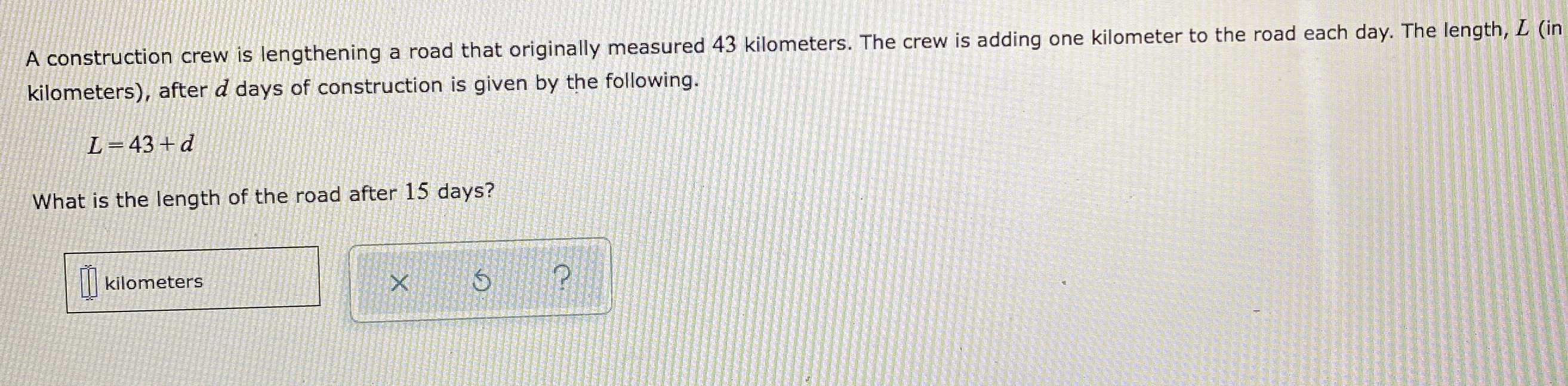### Still have math questions?A construction crew is lengthening a road that originally measured $$43$$ kilometers. The crew is adding one kilometer to the road each day. The length, $$L$$ (in kilometers), after $$d$$ days of construction is given by the following. $$L = 43 + d$$What is the length of the road after $$15$$ days?